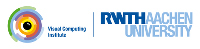OpenMesh
Using STL algorithms

Since the OpenMesh iterators are (almost) conformant to STL iterators, one can apply the STL algorithms on meshes.

The following example shows how to use the STL `for_each` construct, since it is easier to read and may be more efficient than hand-written loops.

We will define a class which provides the smoothing algorithm, hence define a reusable component. The class must be template class because there is no such thing as a class OpenMesh, but many different types of OpenMesh:

template <class Mesh> class SmootherT

The class SmootherT has two functors, one that computes the barycenter for a given vertex, and a second that sets the vertex position to the corresponding barycenter. A functor is simply a class with a function `operator()(...)`. The first functor `ComputeCOG` computes the barycenter and stores it in a custom vertex property `cog_:`

void operator()(const typename Mesh::VertexHandle& _vh)
{
typename Mesh::VertexVertexIter vv_it;
typename Mesh::Scalar valence(0.0);
mesh_.property(cog_, _vh) = typename Mesh::Point(0.0, 0.0, 0.0);
for (vv_it=mesh_.vv_iter(_vh); vv_it.is_valid(); ++vv_it)
{
mesh_.property(cog_, _vh) += mesh_.point( *vv_it );
++valence;
}
mesh_.property(cog_, _vh ) /= valence;
}

Note, that `ComputeCOG` needs to have access to the mesh object and the property handle. Here, both are references to member variables of the smoother object.

The second functor `class` `SetCOG`, which sets the vertex position, is constructed analogical.

Using these functors and `std::for_each` from the STL the smoothing algorithm can be realized in a member function of `SmootherT:`

void smooth(unsigned int _iterations)
{
for (unsigned int i=0; i < _iterations; ++i)
{
std::for_each(mesh_.vertices_begin(),
mesh_.vertices_end(),
ComputeCOG(mesh_, cog_));
std::for_each(mesh_.vertices_begin(),
mesh_.vertices_end(),
SetCOG(mesh_, cog_));
}
}

The complete example looks like this:

#include <algorithm>
#include <OpenMesh/Core/Utils/Property.hh>
#ifndef DOXY_IGNORE_THIS
template <class Mesh> class SmootherT
{
public:
typedef typename Mesh::Point cog_t;
typedef OpenMesh::VPropHandleT< cog_t > Property_cog;
public:
// construct with a given mesh
explicit SmootherT(Mesh& _mesh)
: mesh_(_mesh)
{
}
~SmootherT()
{
mesh_.remove_property( cog_ );
}
// smooth mesh _iterations times
void smooth(unsigned int _iterations)
{
for (unsigned int i=0; i < _iterations; ++i)
{
std::for_each(mesh_.vertices_begin(),
mesh_.vertices_end(),
ComputeCOG(mesh_, cog_));
std::for_each(mesh_.vertices_begin(),
mesh_.vertices_end(),
SetCOG(mesh_, cog_));
}
}
private:
//--- private classes ---
class ComputeCOG
{
public:
ComputeCOG(Mesh& _mesh, Property_cog& _cog)
: mesh_(_mesh), cog_(_cog)
{}
void operator()(const typename Mesh::VertexHandle& _vh)
{
typename Mesh::VertexVertexIter vv_it;
typename Mesh::Scalar valence(0.0);
mesh_.property(cog_, _vh) = typename Mesh::Point(0.0, 0.0, 0.0);
for (vv_it=mesh_.vv_iter(_vh); vv_it.is_valid(); ++vv_it)
{
mesh_.property(cog_, _vh) += mesh_.point( *vv_it );
++valence;
}
mesh_.property(cog_, _vh ) /= valence;
}
private:
Mesh& mesh_;
Property_cog& cog_;
};
class SetCOG
{
public:
SetCOG(Mesh& _mesh, Property_cog& _cog)
: mesh_(_mesh), cog_(_cog)
{}
void operator()(const typename Mesh::VertexHandle& _vh)
{
if (!mesh_.is_boundary(_vh))
mesh_.set_point( _vh, mesh_.property(cog_, _vh) );
}
private:
Mesh& mesh_;
Property_cog& cog_;
};
//--- private elements ---
Mesh& mesh_;
Property_cog cog_;
};
#endif
Handle representing a vertex property.
Definition: Property.hh:417

and

#include <iostream>
#include <vector>
// -------------------- OpenMesh
#include <OpenMesh/Core/IO/MeshIO.hh>
#include <OpenMesh/Core/Mesh/TriMesh_ArrayKernelT.hh>
// --------------------
#include "smooth_algo.hh"
// ----------------------------------------------------------------------------
#ifndef DOXY_IGNORE_THIS
{
};
#endif
// ----------------------------------------------------------------------------
int main(int argc, char **argv)
{
MyMesh mesh;
// check command line options
if (argc != 4)
{
std::cerr << "Usage: " << argv << " #iterations infile outfile\n";
return 1;
}
if ( ! OpenMesh::IO::read_mesh(mesh, argv) )
{
std::cerr << "Error: Cannot read mesh from " << argv << std::endl;
return 1;
}
// smoothing mesh argv times
SmootherT<MyMesh> smoother(mesh);
smoother.smooth(atoi(argv));
// write mesh to stdout
if ( ! OpenMesh::IO::write_mesh(mesh, argv) )
{
std::cerr << "Error: cannot write mesh to " << argv << std::endl;
return 1;
}
return 0;
}
bool write_mesh(const Mesh &_mesh, const std::string &_filename, Options _opt=Options::Default, std::streamsize _precision=6)
Write a mesh to the file _filename.
Definition: MeshIO.hh:190
bool read_mesh(Mesh &_mesh, const std::string &_filename)
Read a mesh from file _filename.
Definition: MeshIO.hh:95
@ PrevHalfedge
Add storage for previous halfedge (halfedges). The bit is set by default in the DefaultTraits.
Definition: Attributes.hh:84
Definition: smooth.cc:9
Triangle mesh based on the ArrayKernel.
Definition: TriMesh_ArrayKernelT.hh:96
Polygonal mesh based on the ArrayKernel.
Definition: PolyMesh_ArrayKernelT.hh:96
Base class for all traits.
Definition: Traits.hh:122

Project OpenMesh, ©  Visual Computing Institute, RWTH Aachen. Documentation generated using doxygen .